In :
import numpy


## 2. Saturation¶

In :
x = numpy.loadtxt('/mnt/saturate/ecoli_5m-report.txt')

In :
plot(x[:,0], x[:,1])
xlabel('number of reads examined')
ylabel('number of reads retained')
title('Saturation of assembly graph/information in E. coli data set')

Out:
<matplotlib.text.Text at 0x7f728c769610>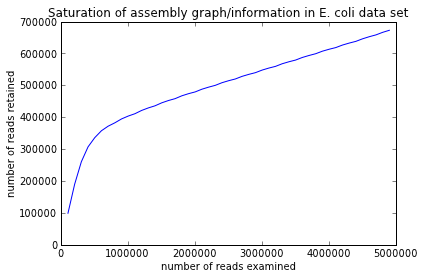## 3. Coverage spectrum - example 1¶

In :
x = numpy.loadtxt('/mnt/cover1/reads.hist')

In :
plot(x[:,0], x[:,1])
xlabel('read abundance')
ylabel('N reads with that abundance')
title('Coverage spectrum of artificial two-species metagenome')

Out:
<matplotlib.text.Text at 0x7f728c443710>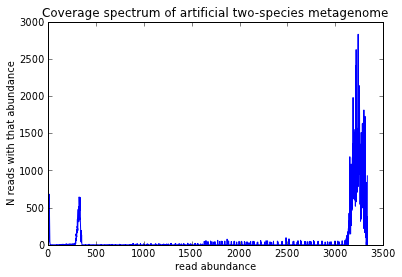## Coverage spectrum -- example 2¶

In :
x = numpy.loadtxt('/mnt/cover2/reads.hist')

In :
plot(x[:,0], x[:,1])
axis(xmax=300)
xlabel('read abundance')
ylabel('N reads with that abundance')
title('Coverage spectrum of E. coli colony')

Out:
<matplotlib.text.Text at 0x7f728c44ce10>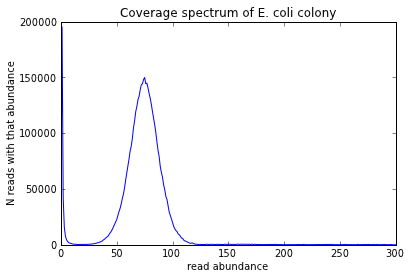## 4. Generating an error profile¶

In :
x = numpy.loadtxt('/mnt/error/ecoli_ref-5m.fastq.gz.errhist')

In :
plot(x[:,0], x[:,2])
xlabel('position in read')
ylabel('number of errors at that position')
title('reference-free error profile for E. coli reads')

Out:
<matplotlib.text.Text at 0x7f728c986a90>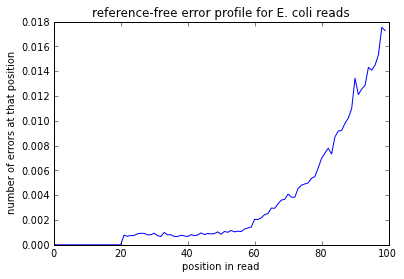## 5. Assembly-filtered k-mer spectrum¶

In :
x = numpy.loadtxt('/mnt/kmercov/counts.out')

In :
plot(x[:,0], x[:,1])
xlabel('k-mer abundance')
ylabel('number of k-mers with that abundance')
title('read-based abundance of k-mers that ended up in assembly')

Out:
<matplotlib.text.Text at 0x465cb10>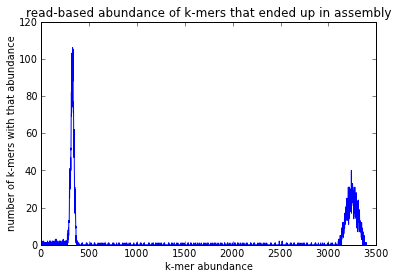## 6. Partitioning¶

In :
x = numpy.loadtxt('/mnt/part/group0.hist')
y = numpy.loadtxt('/mnt/part/group1.hist')

In :
plot(x[:, 0], x[:, 1], label='partition 0')
plot(y[:, 0], y[:, 1], label='partition 1')
axis(ymax=150)
legend()
xlabel('k-mer abundance')
ylabel('number of k-mers with that abundance')
title('partitions correlate with species abundance')

Out:
<matplotlib.text.Text at 0x4684d50>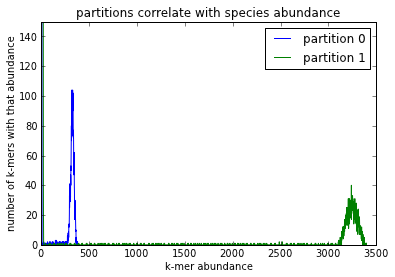In :